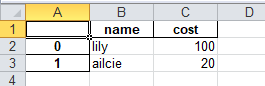• pythonpandas写入excel
2021-12-09 21:44:12

## 写入多个表格

import pandas as pd
import os

if os.path.exists(output_file):
with pd.ExcelWriter(output_file, engine='openpyxl') as excel_writer:
excel_writer.book = book
result_df.to_excel(excel_writer, sheet_name=sheet_name)

else:
result_df.to_excel(output_file, sheet_name=sheet_name)



pd.ExcelWriter()
可选重要参数
mode: ‘w’, ‘a’ write or append default write
测试当mode:'a’时会创建新的sheet 并没有append
if_sheet_exists{‘error’, ‘new’, ‘replace’}, default ‘error’ new in 1.3.0

使用上下文管理器这样就不用担心忘记excel_writer.close()

with pd.ExcelWriter(path, mode='a', engine='openpyxl') as excel_writer:
df.to_excel(excel_writer, sheet_name='1')


多次运行会新建sheet， sheet_name很奇怪,

## 获取excel全部表名

excel = pd.read_excel(sheet_name=None)
excel.keys()

excel = pd.ExcelFile()
excel.sheet_namespython
更多相关内容
• 主要介绍了python pandas写入excel文件的方法示例，文中通过示例代码介绍的非常详细，对大家的学习或者工作具有一定的参考学习价值，需要的朋友们下面随着小编来一起学习学习吧
• 今天小编就为大家分享一篇解决Python pandas df 写入excel 出现的问题，具有很好的参考价值，希望对大家有所帮助。一起跟随小编过来看看吧
• 【文件处理】——Python pandas 写入数据到excel

目录

1、创建一个新的excel表格

2、 获取写入excel的数据data

3、将data类型转换为pandas接受的类型

4、写入到excel中

5、保存excel

最终结果

#!/usr/bin/env python
# -*- coding: utf-8 -*-
# @Time    : 2021/11/9 23:18
# @Author  : @linlianqin
# @Site    :
# @File    : writeDataIntoExcel.py
# @Software: PyCharm
# @description:

import pandas as pd

def writeDataIntoExcel(xlsPath: str, data: dict):
writer = pd.ExcelWriter(xlsPath)
sheetNames = data.keys()  # 获取所有sheet的名称
# sheets是要写入的excel工作簿名称列表
data = pd.DataFrame(data)
for sheetName in sheetNames:
data.to_excel(writer, sheet_name=sheetName)
# 保存writer中的数据至excel
# 如果省略该语句，则数据不会写入到上边创建的excel文件中
writer.save()

if __name__ == '__main__':
data = {"name":["lily","ailcie"],"cost":[100,20]}
xlspath = "3.xls"
writeDataIntoExcel(xlspath,data)


# 1、创建一个新的excel表格

writer = pd.ExcelWriter(xlsPath)

# 2、 获取写入excel的数据data

data的形式是字典的形式，

data = {字段名1：[字段1内容1，字段1内容2,...,字段1内容n],...,字段名n：[字段n内容1，字段n内容2,...,字段n内容n]}

注：字段指的是excel中每一列的列名称

data = {"name":["lily","ailcie"],"cost":[100,20]}

# 3、将data类型转换为pandas接受的类型

data = pd.DataFrame(data)

# 4、写入到excel中

data.to_excel(writer, sheet_name=sheetName)

# 5、保存excel

writer.save()

# 最终结果展开全文python excel 写入数据
• import pandas as pd import numpy as np df = pd.DataFrame(np.arange(12).reshape(3, 4), columns=list('abcd')) excel = pd.ExcelWriter('test.xlsx') df.to_excel(excel, 'aa') sheet = excel.sheets['aa'] ...

报错代码如下：

import pandas as pd
import numpy as np

df = pd.DataFrame(np.arange(12).reshape(3, 4), columns=list('abcd'))
excel = pd.ExcelWriter('test.xlsx')
df.to_excel(excel, 'aa')
sheet = excel.sheets['aa']
sheet.set_column(0, 0, 20)   # 报错：没有此函数


AttributeError: 'Worksheet' object has no attribute 'set_column'

解决方案：

pip install xlsxwriter


参考：

展开全文• pandas读取、写入csv数据非常方便，但是有时希望通过excel画个简单的图表看一下数据质量、变化趋势并保存，这时候csv格式的数据就略显不便，因此尝试直接将数据写入excel文件。 pandas可以写入一个或者工作簿，两种...

pandas读取、写入csv数据非常方便，但是有时希望通过excel画个简单的图表看一下数据质量、变化趋势并保存，这时候csv格式的数据就略显不便，因此尝试直接将数据写入excel文件。

pandas可以写入一个或者工作簿，两种方法介绍如下：

1、如果是将整个DafaFrame写入excel，则调用to_excel()方法即可实现，示例代码如下：

# output为要保存的Dataframe
output.to_excel('保存路径 + 文件名.xlsx')

2、有多个数据需要写入多个excel的工作簿，这时需要调用通过ExcelWriter()方法打开一个已经存在的excel表格作为writer，然后通过to_excel()方法将需要保存的数据逐个写入excel，最后关闭writer。

示例代码如下：

# 创建一个空的excel文件
nan_excle = pd.DataFrame()
nan_excel.to_excel(path + filename)

# 打开excel
writer = pd.ExcelWriter(path + filename)
#sheets是要写入的excel工作簿名称列表
for sheet in sheets:
output.to_excel(writer, sheet_name=sheet)

# 保存writer中的数据至excel
# 如果省略该语句，则数据不会写入到上边创建的excel文件中
writer.save()

示例代码：

pip install xlrd
pip install xlwt
pip install openpyxl

转载于:https://www.cnblogs.com/keeptg/p/10609222.html

展开全文• 将多个Dataframe分别写入Excel的多个Sheets，并且不能覆盖原有的sheet.python
• 0. 前言Python Data Analysis Library 或 pandas 是基于NumPy 的一种工具，该工具是为了解决数据分析任务而创建的。Pandas 纳入了大量库和一些标准的数据模型，提供了高效地操作大型数据集所需的工具，能使我们快速...
• import pandas as pdimport openpyxl#将excel数据读取，输出格式为dataframe格式path = r'/Users/**/PycharmProjects/class/pyclass1/others/file/学生信息表.xlsx'#sheet_name可填写1)excel中表单序号从0开始2)表单...
• 我们时常用pd.to_excel将dataframe写入excel中，只能保留最后一次的，之前的写如的全部被覆盖，搞的一脸懵。defwrtoex(filepath,dict,col)#filepath为文件名的绝对路径，dict为要写入的内容，key为要写入的行号，...python
• Pandaspython的一个数据分析包，纳入了大量库和一些标准的数据模型...一、安装包pandas处理Excel需要xlrd、openpyxl依赖包pip3 install pandaspip3 install xlrdpip3 install openpyxl二、创建Excel写入数据impo...
• 前言在网上找了很多Python处理Excel的方法和代码，都不是很尽人意，所以自己综合网上各位大佬的方法，自己进行了优化，具体的代码如下。博主也是新手一枚，代码肯定有很多需要优化的地方，欢迎各位大佬提出建议~代码...
• import os,pandas as pd,re#1.获取文件夹下要合并的文件名dirpath = '文件夹地址'#工作表3特殊处理 需要开始下标和结束下标begin = 231end = 238excel_names = os.listdir(dirpath)#2.获取文件内容sheet_1_merge = ...python
• Python pands读取Excel 数据写入到数据库python 开发语言
• pandas写入excelIn this tutorial we will learn how to work with Excel files and Python. It will provide an overview of how to use Pandas to load and write these spreadsheets to Excel. In the first ...列表 python java 大数据 人工智能
• 读写excel需要用到： pip install xlrd -i https://pypi.tuna.tsinghua.edu.cn/simple pip install xlwt -i https://pypi.tuna.tsinghua.edu.cn/simple 示例代码： import numpy as np import pandas as pd df1 = ...python excel
• python pandas 如何读取Excel数据并重新按照指定格式写入Excel文件呢？ 说一下个人常见的用法心得： 常用且实用的方法： (注意：使用下列方法，记得导入如下库) import pandas as pd import ast 1.读取Excel...python
•python 数据挖掘
• 将爬取的数据填入指定的单元格，而不需要更改Excel格式的情况，或是将一个Excel指定单元格内容复制后插入另一个Excel的单元格。python 开发语言
• df1 = pd.read_excel('pandas_test.xlsx') 2、字典方式创建数据表 （columns参数不仅可以提供字段名，还可以确定字段顺序） df2 = pd.DataFrame({"name":["Tom","Marry","Luse"], "age":[18,19,20], "enjoy":[...python 开发语言...